SPSS TUTORIALS BASICS ANOVA REGRESSION FACTOR CORRELATION

# SPSS Wilcoxon Signed-Ranks Test – Simple Example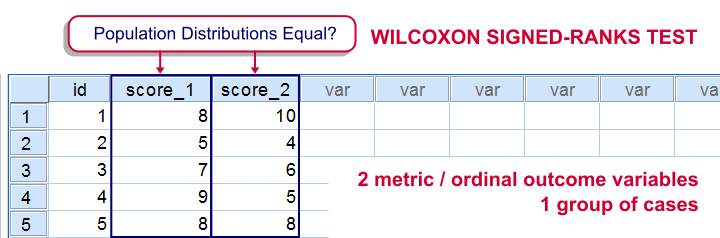For comparing two metric variables measured on one group of cases, our first choice is the paired-samples t-test. This requires the difference scores to be normally distributed in our population. If this assumption isn't met, we can use Wilcoxon S-R test instead. It can also be used on ordinal variables -although ties may be a real issue for Likert items.
Don't abbreviate “Wilcoxon S-R test” to simply “Wilcoxon test” like SPSS does: there's a second “Wilcoxon test” which is also known as the Mann-Whitney test for two independent samples.

## Wilcoxon Signed-Ranks Test - How It Basically Works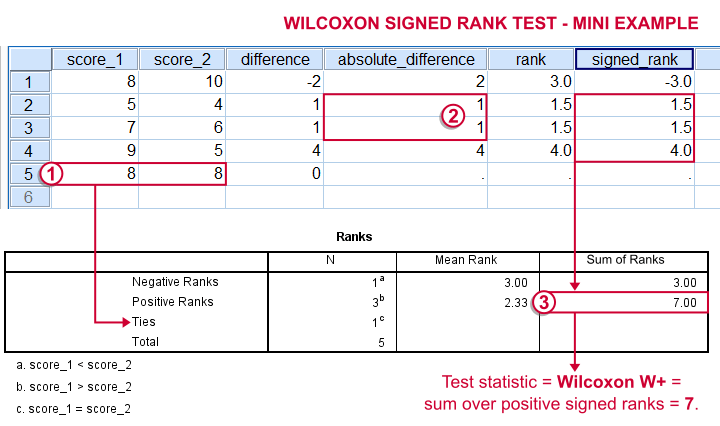1. For each case calculate the difference between score_1 and score_2.Ties (cases whose two values are equal) are excluded from this test altogether.
2. Calculate the absolute difference for each case.
3. Rank the absolute differences over cases.Use mean ranks for ties (different cases with equal absolute difference scores).
4. Create signed ranks by applying the signs (plus or minus) of the differences to the ranks.
5. Compute the test statisticWilcoxon W+, which is the sum over positive signed ranks. If score_1 and score_2 really have similar population distributions, then W+ should be neither very small nor very large.
6. Calculate the p-value for W+ from its exact sampling distribution or approximate it by a standard normal distribution.

So much for the theory. We'll now run Wilcoxon S-R test in SPSS on some real world data.

## Adratings Data - Brief Description.

A car manufacturer had 18 respondents rate 3 different commercials for one of their cars. They first want to know which commercial is rated best by all respondents. These data -part of which are shown below- are in adratings.sav.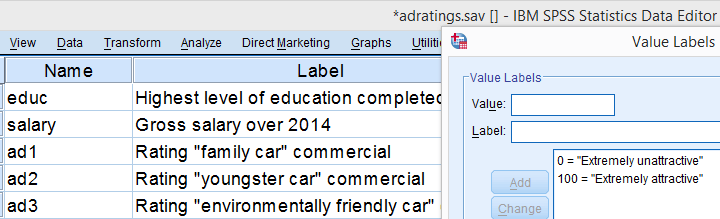## Quick Data Check

Our current focus is limited to the 3 rating variables, ad1 through ad3. Let's first make sure we've an idea what they basically look like before carrying on. We'll inspect their histograms by running the syntax below.

## Basic Histograms Syntax

*Check if data look plausible with histograms.

/format notable
/histogram.

## Histograms - Results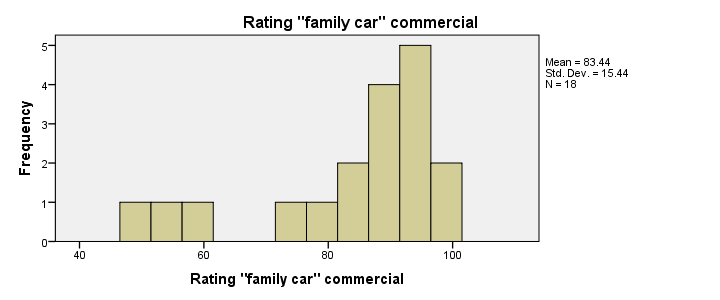First and foremost, our 3 histograms don't show any weird values or patterns so our data look credible and there's no need for specifying any user missing values.
Let's also take a look at the descriptive statistics in our histograms. Each variable has n = 18 respondents so there aren't any missing values at all. Note that ad2 (the “Youngster car commercial”) has a very low average rating of only 55. It's decided to drop this commercial from the analysis and test if ad1 and ad3 have equal mean ratings.

## Difference Scores

Let's now compute and inspect the difference scores between ad1 and ad3 with the syntax below.

*Compute difference scores.

*Inspect histogram difference scores for normality.

frequencies diff
/format notable
/histogram normal.

## ResultOur first choice for comparing these variables would be a paired samples t-test. This requires the difference scores to be normally distributed in our population but our sample suggests otherwise. This isn't a problem for larger samples sizes (say, n > 25) but we've only 18 respondents in our data.For larger sample sizes, the central limit theorem ensures that the sampling distribution of the mean will be normal, regardless of the population distribution of a variable. Fortunately, Wilcoxon S-R test was developed for precisely this scenario: not meeting the assumptions of a paired-samples t-test. Only now can we really formulate our null hypothesis: the population distributions for ad1 and ad3 are identical. If this is true, then these distributions will be slightly different in a small sample like our data at hand. However, if our sample shows very different distributions, then our hypothesis of equal population distributions will no longer be tenable.

## Wilcoxon S-R test in SPSS - Menu

Now that we've a basic idea what our data look like, let's run our test. The screenshots below guide you through.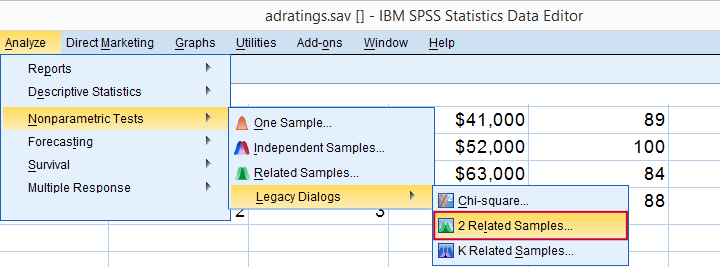2 Related Samples refers to comparing 2 variables measured on the same respondents. This is similar to “paired samples” or “within-subjects” effects in repeated measures ANOVA.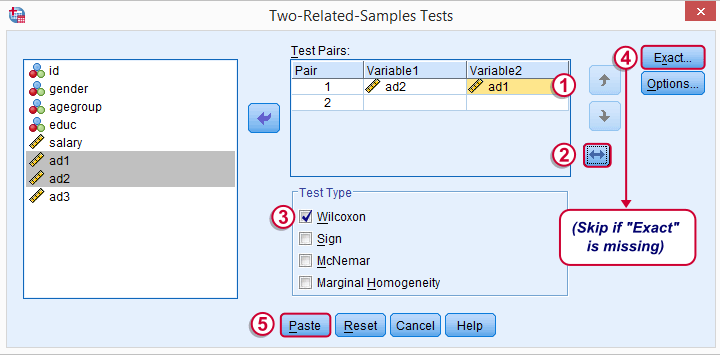Optionally, reverse the variable order so you have the highest scores (ad1 in our data) under Variable2.“Wilcoxon” refers to Wilcoxon S-R test here. This is a different test than Wilcoxon independent samples test (also know as Mann-Whitney test).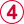may or may not be present, depending on your SPSS license. If you do have it, we propose you fill it out as below.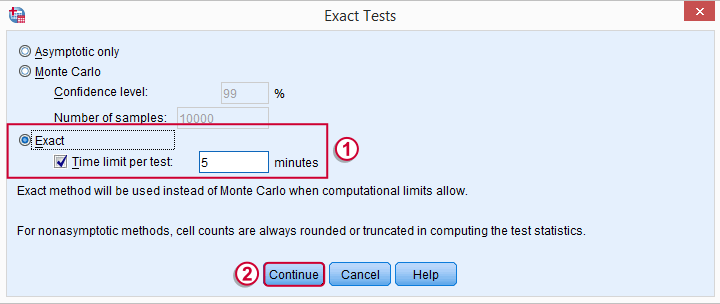## Wilcoxon S-R test in SPSS - Syntax

Following these steps results in the syntax below (you'll have one extra line if you requested exact statistics).

*Wilcoxon Signed Ranks Test Syntax.

NPAR TESTS
/MISSING ANALYSIS.

## Wilcoxon S-R Test - Ranks Table Ouput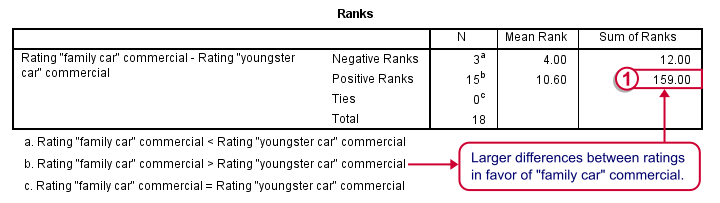Let's first stare at this table and its footnotes for a minute and decipher what it really says. Right. Now, if ad1 and ad3 have similar population distributions, then the signs (plus and minus) should be distributed roughly evenly over ranks. If you find this hard to grasp -like most people- take another look at this diagram.
This implies that the sum of positive ranks should be close to the sum of negative ranks. This number (159 in our example) is our test statistic and known asWilcoxon W+.
Our table shows a very different pattern: the sum of positive ranks (indicating that the “Family car” was rated better) is way larger than the sum of negative ranks. Can we still believe our 2 commerials are rated similarly?

## Wilcoxon S-R Test - Test Statistics Ouput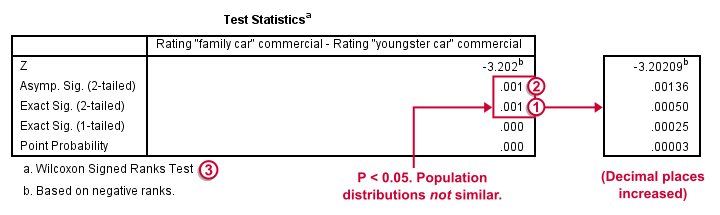Oddly, our ”Test Statistics“ table includes everything except for our actual test statistic, the aforementioned W+.We prefer reporting Exact Sig. (2-tailed). Its value of 0.001 means that the probability is roughly 1 in 1,000 of finding the large sample difference we did if our variables really have similar population distributions.If our output doesn't include the exact p-value, we'll report Asymp. Sig. (2-tailed) instead, which is also 0.001. This approximate p-value is based on the standard normal distribution (hence the “Z” right on top of it).“Asymp” is short for asymptotic. It means that as the sample size approaches infinity, the sampling distribution of W+ becomes identical to a normal distribution. Or more practically: this normal approximation is more accurate for larger sample sizes.
It's comforting to see that both p-values are 0.001. Apparently, the normal approximation is accurate. However, if we increase the decimal places, we see that it's almost three times larger than the exact p-value.A nice tool for doing so is downloadable from Set Decimals for Output Tables Tool.
The reason for having two p-values is that the exact p-value can be computationally heavy, especially for larger sample sizes.

## How to Report Wilcoxon Signed-Ranks Test?

The official way for reporting these results is as follows: “A Wilcoxon Signed-Ranks test indicated that the “Family car” commercial (mean rank = 10.6) was rated more favorably than the “Youngster car” commercial (mean rank = 4.0), Z = -3.2, p = 0.001.” We think this guideline is poor for smaller sample sizes. In this case, the Z-approximation may be unnecessary and inaccurate and the exact p-value is to be preferred.

I hope this tutorial has been helpful in understanding and using Wilcoxon Signed-Ranks test in SPSS. Please let me know by leaving a comment below. Thanks!

# Tell us what you think!

*Required field. Your comment will show up after approval from a moderator.

# THIS TUTORIAL HAS 29 COMMENTS:

•### By Jacq on November 16th, 2019

•### By Taner on December 13th, 2020

What is the "z" formulation in SPSS?

•### By nav on April 19th, 2021

Thank you so much!!!! very helpful compared to all the other guides out there

•### By Babitha E K,Asst.Professor on August 31st, 2021

sir
if i am checking the effect of treatment in relation to pre test and post test score for treatment and control group
i did the sameway as this tutorial and i got for treament pretest and post-test negative rank meanrank as 28.5 and 0.00 as positive rank . how i will interpret it